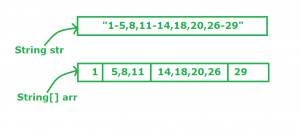# Java program to expand a String if range is given?

Suppose we have given a String in which some ranges as specified and we have to place the numbers which is between the given range in the specified place as given in the example:

Examples:

```Input : string x = "1-5, 8, 11-14, 18, 20, 26-29"
Output : string y = "1, 2, 3, 4, 5, 8, 11, 12,
13, 14, 18, 20, 26, 27, 28, 29"
```

Approach: To solve the above problem, we can follow the below approach:

• First we have to split the String into String[] array. We have to split the String where we found – symbol.
•• Now we have String[] array with the elements. Now we just go to the first index last element i.e. 1 and preceding index first element of the String[] array i.e. 5 .
• After that by the help of for loop we can add the numbers which is between 1 and 5 and store it in the String variable.
• The above process continue til the length of String[] array.

NOTE: By the help of Collections and various utility methods we can solve the problem easily but Collections concept is not good option performance wise. If we go through Collections, performance is reduced and time complexity is also increased.There in the below program we explicitly define our own split method and logic.

 `// Java code to demonstrate expansion ` `// of string ` ` `  `public` `class` `StringExpand { ` ` `  `    ``static` `String[] split(String st) ` `    ``{ ` `        ``// Count how many words in our string ` `        ``// Irrespective of spaces ` `        ``int` `wc = countWords(st); ` `        ``String w[] = ``new` `String[wc]; ` `        ``char``[] c = st.toCharArray(); ` `        ``int` `k = ``0``; ` `        ``for` `(``int` `i = ``0``; i < c.length; i++) { ` `            ``String s = ``""``; ` ` `  `            ``// Whenever we found an non-space character ` `            ``while` `(i < c.length && c[i] != ``' '``) { ` `                ``// Concat with the String s ` `                ``// Increment the value of i ` `                ``s = s + c[i]; ` `                ``i++; ` `            ``} ` ` `  `            ``// If the String is not empty ` `            ``if` `(s.length() != ``0``) { ` ` `  `                ``// Add the String to the String[] ` `                ``// array ` `                ``w[k] = s; ` `                ``k++; ` `            ``} ` `        ``} ` ` `  `        ``// Returning the String[] array ` `        ``return` `w; ` `    ``} ` ` `  `    ``static` `int` `countWords(String str) ` `    ``{ ` `        ``int` `count = ``0``; ` `        ``for` `(``int` `i = ``0``; i < str.length(); i++) { ` ` `  `            ``// The below condition to check ` `            ``// whether the first character is ` `            ``// space or not ` `            ``if` `(i == ``0` `&& str.charAt(i) != ``' '` `|| str.charAt(i) != ``' '` `&& str.charAt(i - ``1``) == ``' '``) { ` `                ``count++; ` `            ``} ` `        ``} ` `        ``return` `count; ` `    ``} ` ` `  `    ``public` `static` `void` `expand(String s) ` `    ``{ ` `        ``String p = s; ` `        ``String[] arr = p.split(``"\\-"``); ` `        ``String k = ``""``; ` `        ``for` `(``int` `i = ``0``; i < arr.length; i++) { ` `            ``if` `(i != arr.length - ``1``) { ` `                ``String[] arr1 = arr[i].split(``", "``); ` `                ``String[] arr2 = arr[i + ``1``].split(``", "``); ` `                ``int` `a = Integer.parseInt(arr1[arr1.length - ``1``]); ` `                ``int` `b = Integer.parseInt(arr2[``0``]); ` `                ``for` `(``int` `j = a + ``1``; j < b; j++) { ` `                    ``arr[i] = arr[i] + ``", "` `+ j; ` `                ``} ` `            ``} ` `            ``if` `(k != ``""``) ` `                ``k = k + ``", "` `+ arr[i]; ` `            ``else` `                ``k = k + arr[i]; ` `        ``} ` ` `  `        ``System.out.println(k); ` `    ``} ` ` `  `    ``// Driver code ` `    ``public` `static` `void` `main(String[] args) ` `    ``{ ` `        ``String s = ``"1-5, 8, 11-14, 18, 20, 26-29"``; ` `        ``expand(s); ` `    ``} ` `} `

Output:

```1, 2, 3, 4, 5, 8, 11, 12, 13, 14, 18, 20, 26, 27, 28, 29
```

Simpler Implementation :

 `public` `class` `Solution { ` ` `  `    ``public` `static` `void` `expand(String word) ` `    ``{ ` ` `  `        ``StringBuilder sb = ``new` `StringBuilder(); ` ` `  `        ``// Get all intervals ` `        ``String[] strArr = word.split(``", "``); ` ` `  `        ``// Traverse through every interval ` `        ``for` `(``int` `i = ``0``; i < strArr.length; i++) { ` ` `  `            ``// Get lower and upper ` `            ``String[] a = strArr[i].split(``"-"``); ` ` `  `            ``if` `(a.length == ``2``) { ` ` `  `                ``int` `low = Integer.parseInt(a[``0``]); ` `                ``int` `high = Integer.parseInt(a[a.length - ``1``]); ` ` `  `                ``// Append all numbers ` `                ``while` `(low <= high) { ` `                    ``sb.append(low + ``" "``); ` `                    ``low++; ` `                ``} ` `            ``} ` `            ``else` `{ ` `                ``sb.append(strArr[i] + ``" "``); ` `            ``} ` `        ``} ` ` `  `        ``System.out.println(sb.toString()); ` `    ``} ` ` `  `    ``public` `static` `void` `main(String args[]) ` `    ``{ ` `        ``String s = ``"1-5, 8, 11-14, 18, 20, 26-29"``; ` `        ``expand(s); ` `    ``} ` `} `

Output:

```1 2 3 4 5 8 11 12 13 14 18 20 26 27 28 29
```

Don’t stop now and take your learning to the next level. Learn all the important concepts of Data Structures and Algorithms with the help of the most trusted course: DSA Self Paced. Become industry ready at a student-friendly price.

My Personal Notes arrow_drop_upIf you like GeeksforGeeks and would like to contribute, you can also write an article using contribute.geeksforgeeks.org or mail your article to contribute@geeksforgeeks.org. See your article appearing on the GeeksforGeeks main page and help other Geeks.

Please Improve this article if you find anything incorrect by clicking on the "Improve Article" button below.

Improved By : rahulkumarverma0507

Article Tags :
Practice Tags :

2

Please write to us at contribute@geeksforgeeks.org to report any issue with the above content.